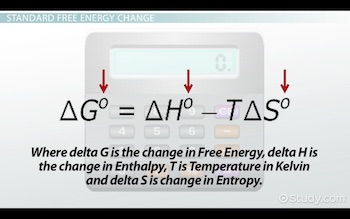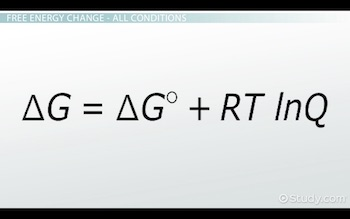# The Relationship Between Free Energy and the Equilibrium Constant

An error occurred trying to load this video.

Try refreshing the page, or contact customer support.

Coming up next: Matter & Experimental Chemistry Flashcards

### You're on a roll. Keep up the good work!

Replay
Your next lesson will play in 10 seconds
• 0:01 Standard Free Energy Change
• 3:31 Free Energy Change -…
• 4:45 Free Energy & the…
• 6:52 Calculating the…
• 8:26 Lesson Summary
Save Save

Want to watch this again later?

Timeline
Autoplay
Autoplay
Speed Speed Audio mode

#### Recommended Lessons and Courses for You

Lesson Transcript
Instructor: Nicola McDougal

Nicky has taught a variety of chemistry courses at college level. Nicky has a PhD in Physical Chemistry.

In this lesson, we learn the important connection between free energy and the equilibrium constant. We will begin by considering systems under non-standard conditions to derive the relationship. We will see how to relate the free energy change to the extent of a chemical reaction.

## Standard Free Energy Change

Say you go into a soda store in London and ask for their standard sized can of cola. You would assume that the can size would be the same as a standard can of cola you bought in the USA. Imagine your surprise when the London can is almost 10% smaller.

Huh, so much for standard. In this case, a standard size is not really a standard at all. You are not sure what to expect when you ask for a standard can of cola in different countries.

In science and chemistry, we need to be more exact. When we say standard, we need everyone around the world to know exactly what standard means. Standards are defined precisely.

Okay, so let us consider this a little further with our thermodynamics hat on. You may recall that Gibbs free energy tells us whether a reaction is spontaneous or not. When we calculate the Gibbs free energy change of a reaction, we often use this equation:

delta G^0 = delta H^0 - T delta S^0

Where delta G is the change in free energy, delta H is the change in enthalpy, T is temperature in Kelvin and delta S is change in entropy.

Notice the little degree signs on each of the letters:These indicate that we are at standard conditions. Therefore, we are measuring the standard free energy change: delta G naught or delta G standard.

In thermodynamics, standard conditions are gases at one atmosphere partial pressure and ions or molecules in solution at one molar concentration. It is important to realize that temperature is not included as a standard condition. However, the thermodynamic values at 25 degrees celsius are usually found in data tables.

When we measure delta G standard, we can find out whether the reaction is spontaneous at standard conditions. The general equation is A + B forms C + D.

If delta G standard is negative, the reaction is spontaneous at standard conditions. In other words, the reaction will spontaneously move forward to form products. If delta G standard is positive, the reaction is non-spontaneous at standard conditions. In other words, the reaction will not move forward to form products; instead, the reverse reaction is spontaneous.

If delta G standard is zero, the system is at equilibrium at standard conditions. This time the rate of the forward and reverse reaction is the same, and the system is at equilibrium. There is no tendency for the reaction to go in either direction.

So, to help you keep these straight, remember this little phrase: Naughty Forensic Physicists Remember Zero Equations, which is, if delta G standard is Negative, the reaction moves Forward. If it is Positive, the reaction moves in Reverse. When delta G standard equals Zero, the reaction is at Equilibrium.

## Free Energy Change - All Conditions

Now, it is true that we often work at standard conditions so we can just work out the standard free energy change. But it is helpful to define an equation that allows us to calculate the free energy change at all conditions, in particular, the concentration condition, as we are normally always at standard pressure conditions.

At all conditions, it turns out we have the following relationship:

delta G = delta G^0 + RT ln Q

Here, delta G at any condition is equal to delta G standard plus the gas constant R, multiplied by temperature (T) in Kelvin, multiplied by the natural log of the reaction quotient. Notice the little degree sign has disappeared on the first delta G:The reaction quotient (Q) measures the relative amounts of products and reactants present during a reaction at a particular point in time.

Now, we are not going to use this equation to do calculations in this lesson. But instead, we use it to make the connection between free energy and another very important quantity, the equilibrium constant.

## Free Energy and the Equilibrium Constant

The equilibrium constant (K) is another way we can tell if a reaction is spontaneous. So let us see how it is related to free energy. Recall that Q tells us the ratio of products to reactants in a reaction mixture. It is also true that when a reaction is at equilibrium, then Q = K. And finally, we have also just learned that equilibrium delta G = 0.

By substituting all this information into our equation, we can now see the following relationship:

0 = delta G^0 + RT ln K

So, put more simply, we end up with:

delta G^0 = - RT ln K

Here, delta G standard is equal to negative R, the gas constant, multiplied by the temperature in Kelvin, multiplied by the natural log of the equilibrium constant.

To unlock this lesson you must be a Study.com Member.

### Register to view this lesson

Are you a student or a teacher?

#### See for yourself why 30 million people use Study.com

##### Become a Study.com member and start learning now.
Back
What teachers are saying about Study.com

### Earning College Credit

Did you know… We have over 200 college courses that prepare you to earn credit by exam that is accepted by over 1,500 colleges and universities. You can test out of the first two years of college and save thousands off your degree. Anyone can earn credit-by-exam regardless of age or education level.